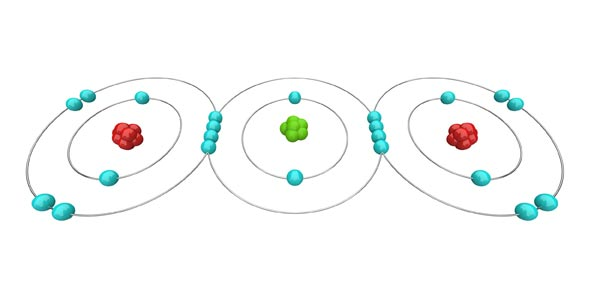# Ionic And Covalent Bonds (Pat Naylor)

10 Questions | Total Attempts: 43SettingsQuiz about ionic and covalent bonds.

Related Topics
• 1.
What is a covalent bond?
• A.

A type of chemical bond that involves a metal and a nonmetal ion through electrostatic attraction

• B.

A form of chemical bonding that is characterized by the sharing of pairs of electrons between atoms.

• C.

A bond between 3 or more molecules

• D.

None of the Above

• 2.
What is an ionic bond?
• A.

A form of chemical bonding that is characterized by the sharing of pairs of electrons between atoms, and other covalent bonds

• B.

A bond between elements that form ions

• C.

A type of chemical bond that involves a metal and a nonmetal ion through electrostatic attraction

• D.

None of the above

• 3.
What type of bond is this?
• A.

Single Ionic Bond

• B.

Triple Bond

• C.

Double Covalent Bond

• D.

Oxygen

• 4.
What type of bond is this?
• A.

Double Bond

• B.

Triple Bond

• C.

Single Bond

• D.

None of the Above

• 5.
And this type of bond is______.
• A.

Single Bond

• B.

Hydrogen

• C.

Electron Sea Model Bond

• D.

Double Bond

• E.

Triple Bond

• 6.
What is the strongest type of covalent chemical bond?
• A.

Pi Bond

• B.

Sigma Bond

• C.

There isn't any

• D.

Double Bond

• E.

Triple Bond

• 7.
___________ are covalent chemical bonds where two lobes of one involved electron orbital overlap two lobes of the other involved electron orbital.
• A.

Sigma Bonds

• B.

Powerful Bonds

• C.

Pi Bonds

• D.

Triple Bonds

• E.

Double Bonds

• 8.
Sodium Chloride (NaCl) is an example of what type of bond?
• A.

Covalent Bond

• B.

Triple Bond

• C.

Double Bond

• D.

Metallic Bond

• E.

Ionic Bond

• 9.
H           H    C  =  C H           H(ethene)This is an example of what type of bond?
• A.

Double Covalent Bond

• B.

Triple Covalent Bond

• C.

Ionic Bond

• D.

None of the Above

• 10.
If Lithium bonded with Fluorine, what type of bond would occur?
• A.

Covalent Bond

• B.

Organic Bond

• C.

Pi Bond

• D.

Sigma Bond

• E.

Ionic Bond